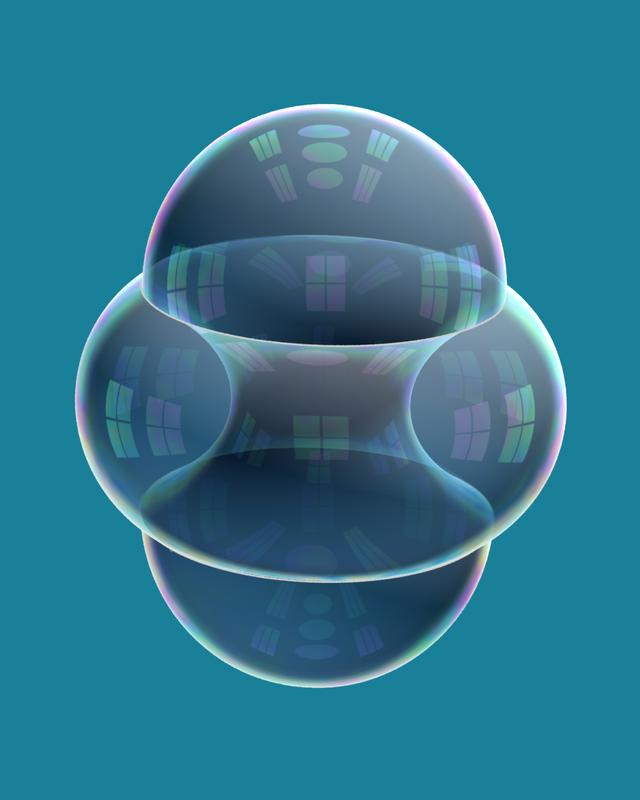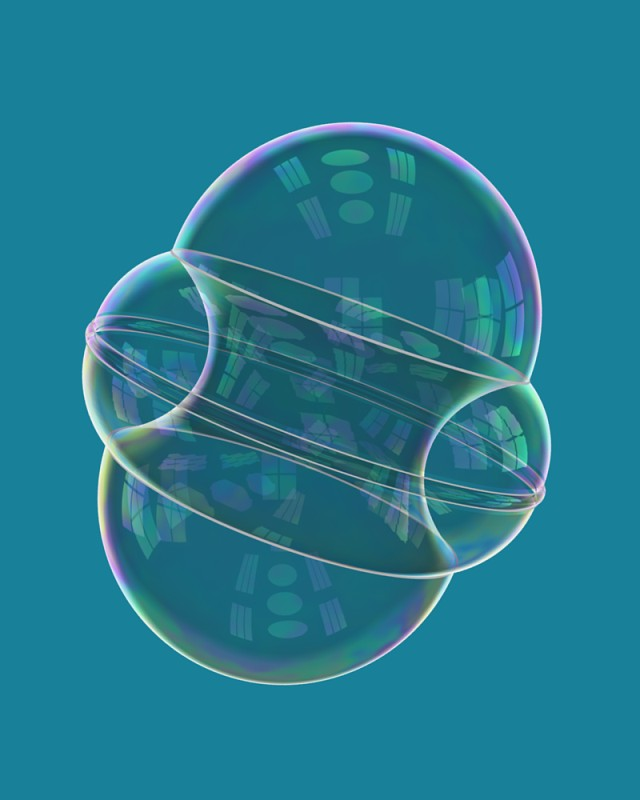# Soap bubbles and isoperimetric problems

Because of surface tension, soap bubbles or clusters thereof naturally try to minimize area for the volume(s) they enclose. This suggests the following mathematical questions. The isoperimetric problem, in an n-dimensional Riemannian manifold, is to enclose a region of a given (n-dimensional) volume v using a hypersurface of the smallest possible "area" (n-1 dimensional volume). For example, in Euclidean space, a classical theorem asserts that the unique area-minimizer is a sphere. The "soap bubble problem" is a generalization in which the problem is to enclose and separate m regions of prescribed volumes v_1, ..., v_m, using a (singular) hypersurface of minimal area. In many cases there is a natural conjecture for the area-minimizer, but proving it can be extremely difficult! For example, the Double Bubble Conjecture in R^3 was proved only recently. This conjecture, or now theorem, asserts that the least-area enclosure of two prescribed volumes in R^3 is the "standard double bubble", consisting of three pieces of spheres meeting along a circle at 120 degree angles. There is one such shape for each pair of volumes; if the two volumes are equal, the middle "sphere" is a flat disc. The standard double bubble looks like this:The typical strategy for trying to prove something like this is to assume that you have an area-minimizing double bubble, and show that if it is nonstandard then you can modify it to decrease area. The first difficulty is proving that an area-minimizing surface exists! This is not at all trivial, and was accomplished by Almgren in the 1970's. Also, to get an existence theorem you generally have to enlarge the space of surfaces under consideration to include some "bad" surfaces that you don't like, and then struggle to rule these out. In particular, we cannot a priori guarantee that the enclosed regions, or the exterior region, will be connected. This difficulty is partially addressed below.

The triple bubble problem in R^3 currently seems hopeless without some brilliant new idea, although again there is a natural candidate surface. Indeed there are standard enclosures of m volumes in R^n for m &le n+1, given by stereographic projections of regular simplices in spheres.

Anyway, here are some papers on this subject.

•  The shortest enclosure of three connected areas in R^2 (with C. Cox, L. Harrison, S. Kim, J. Light, A. Mauer, and M. Tilton),
Real Analysis Exchange 20 (1994-95), 313-35. pdf
Given three prescribed areas A_1, A_2, A_3, we determine the shortest union of curves in R^2 whose complement has four connected components, three of which are bounded and have areas A_1,A_2,A_3. This is a generalization to multiple regions of the classical isoperimetric theorem. We introduce the ``overlapping bubbles'' trick, with which we can rule out some pathological competitors by temporarily allowing the curves to cross each other.

This paper is based on work done at a Research Experiences for Undergraduates program at Williams College under the guidance of Frank Morgan. I highly recommend a program like this for any undergraduate seriously considering a career in mathematics.

•  The structure of area-minimizing double bubbles,
Journal of Geometric Analysis 7 (1997), 285-304. Postscript.
This paper investigates how to enclose and separate two (possibly disconnected) regions of prescribed volumes in R^n, S^n, or H^n with the least possible surface area. We prove that the exterior region must be connected, and we develop techniques to bound the number of components of the enclosed regions in an area-minimizer. The key is a topological argument which proves that the least area required to enclose two volumes is a concave function of the two volumes. We also prove a general symmetry theorem for minimal enclosures of m volumes in R^n for m < n, based on an idea due to Brian White.

•  The double bubble conjecture (with J. Hass and R. Schlafly),
Electronic Research Announcements of the AMS 1 (1995), 98-102. Postscript PDF
The topological complexity bounds of , together with known symmetry results, reduce the isoperimetric problem for two volumes in R^n to a finite-dimensional minimization problem. Using rigorous numerical methods, Hass and Schlafly solved this problem for two equal volumes in R^3, showing that the unique minimizer is the ``standard double bubble''. Article  is a joint announcement of our results.

•  The isoperimetric problem on surfaces of revolution of decreasing Gauss curvature (with H. Howards and F. Morgan),
Transactions of the AMS 352 (2000), 4889-4909. Postscript PDF
We solve the problem described by the title.

•  Proof of the double bubble conjecture (with F. Morgan, M. Ritore, and A. Ros),
Electronic Research Announcements of the AMS 6 (2000), 45-49. Postscript PDF

•  Proof of the double bubble conjecture (with F. Morgan, M. Ritore, and A. Ros),
Annals of Mathematics 155 (2002), no. 2, 459-489. pdf
This paper proves that the standard double bubble in R^3 is uniquely area-minimizing for arbitrary volumes, without the help of a computer. (Compare .) The key is a new instability argument, which rules out the nonstandard possibilities that were not already ruled out by . They look like this:Some further developments: Many additional results on the double bubble problem in R^n, S^n, and H^n, and other spaces have been proved by various groups of undergraduates working with Frank Morgan. For an excellent introduction to this whole subject I recommend his book, Geometric measure theory: a beginner's guide, third edition. In 2002, W. Wichiramala solved the triple bubble problem in R^2. (Paper  above only solved the easier version of this problem in which the enclosed and exterior regions are assumed to be connected.)

To demonstrate our ignorance, here are three embarassingly simple open problems.

• Is the standard double bubble the only stable (i.e. locally area-minimizing) enclosure of two connected regions in R^3? This would explain the physical fact that only standard double bubbles are observed in real life (when you blow two soap bubbles stuck together).

• Suppose you try to solve the isoperimetric problem or the soap bubble problem in a product of compact Riemannian manifolds M x N, where the metric on N is rescaled to be very small with respect to M and the volumes that you want to enclose. Is every solution the product of a solution on M with all of N?

• Let D be a compact subset of R^2 of area pi, say with smooth boundary. Suppose you want to enclose area A in D using the shortest possible curve or union of curves (where you can use the boundary of D for free). Conjecture (Wicharamala): the length of the shortest possible curve is less than or equal to the corresponding length when D is the unit disk, with equality only if D is the unit disk.

The pictures above are copyright John Sullivan.

You are vistor number to this page since my web counters got reset.